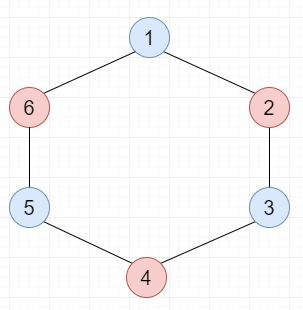# Check if a given graph is Bipartite using DFS in C++ program

C++Server Side ProgrammingProgramming

Suppose we have a connected graph; we have to check whether the graph is bipartite or not. If the graph coloring is possible applying two colors such that nodes in a set are colored with the same color.

So, if the input is likethen the output will be True

To solve this, we will follow these steps −

• Define a function insert_edge(), this will take an edge array adj, u, v,
• insert v at the end of adj[u]
• insert u at the end of adj[v]
• From the main method do the following,
• for each u in adj[v],do
• if visited[u] is same as false, then −
• visited[u] := true
• color[u] := invert of color[v]
• if not is_bipartite_graph(adj, u, visited, color), then −
• return false
• otherwise when color[u] is same as color[v], then −
• return false
• return true

## Example (C++)

Let us see the following implementation to get better understanding −

Live Demo

#include <bits/stdc++.h>
using namespace std;
void insert_edge(vector<int> adj[], int u, int v){
}
bool is_bipartite_graph(vector<int> adj[], int v, vector<bool>& visited, vector<int>& color){
for (int u : adj[v]) {
if (visited[u] == false) {
visited[u] = true;
color[u] = !color[v];
return false;
}
else if (color[u] == color[v])
return false;
}
return true;
}
int main() {
int N = 6;
vector<bool> visited(N + 1);
vector<int> color(N + 1);
visited = true;
color = 0;
cout << (is_bipartite_graph(adj_list, 1, visited, color));
}

## Input

insert_edge(adj_list, 1, 2);
insert_edge(adj_list, 6, 1);
1